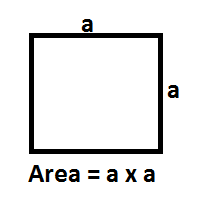# Area of a Square Formula

In geometry, a Square is a flat figure or shape which has four equal sides and every angle is a right angle, i.e. 90°. From this, it follows that the opposite sides are also parallel. It is also described as a rectangle which has two adjacent sides have equal length.  The area of a square can be found by measuring the amount of space occupied within the square. Below mentioned is the formula to calculate the area of a square. It is one of the known type of quadrilaterals in geometry. A square is simply a specific case of a regular polygon, with 4 equal sides. All the facts and properties described for regular polygons can also be applied to a square.$\LARGE Area\:of\:a\:Square\:Formula=a^{2}$
Where,
a is the length of the side of a square

### Solved Examples

Question 1: Find the area of the square of side 16 cm.
Solution:

Side of the square = a = 16 cm
Area of the square
= a2
= 162 cm2
= 256 cm2
Question 2: Find the length of the square whose area is 529 cm2.
Solution:
Area of the square = A = 529 cm2
Side of the square = a =?

Area of the square = A = a2
⇒ 529 cm= a
⇒ a =
$$\begin{array}{l}\sqrt{529}\end{array}$$
⇒ a = 23 cm
Hence, the length of the side of this square is 23 cm.